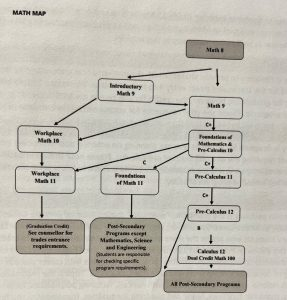Students obtaining less than a C+ in Math 9, or Foundations of Math & Pre-Calculus 10, or Pre-Calculus 11 have a risk of failure at the next grade level. It is recommended that these students discuss the options with their counsellors including the possibility of repeating the course or switching pathways.

Important Note to Students Entering Grade 10 Math: EMCS offers two courses at the Grade 10 level representing the two math pathways through high school. Your choices are Workplace Math 10 or Foundations of Mathematics and Pre-Calculus 10. Please read the details for these courses as well as the following general descriptions of the two pathways.

Important Note to Students Entering Grade 9 Math: EMCS offers two courses at the Grade 9 level. Students who are not confident entering Math 9 directly should choose to take Introductory Math as an additional course.

Mathematics 9 (MMA–09)

Mathematics 9 is a required course which covers a wide range of topics. The skills covered in mathematics 9 and 10 become the foundation upon which the senior mathematics courses are built. Success in future mathematics courses is largely dependent on a solid understanding of the concepts of mathematics 9. Topics: operations with rational numbers, exponents, linear relations, proportional reasoning, statistics and financial literacy.

Introductory Mathematics 9 (XLDCB09IMA)

Introductory Mathematics 9 is a course designed for students who had some difficulties in Math 8 and need to solidify their basic skills before proceeding to Mathematics 9. Introductory Mathematics 9 reinforces many of the concepts developed in Math 8 (integer operations, fractions, decimals, order of operations) and introduces material to prepare the student for Mathematics 9 (rational expressions, algebra and geometry). Reading comprehension is a portion of this course and will form part of the assignments. This course is offered in the first semester so that students can take Mathematics 9, or some students may proceed to Workplace Math 10 by special arrangement with their counselor. It is strongly recommended that students who had difficulty with Math 8 take Introductory Mathematics 9. Required Equipment: Scientific Calculator.

Workplace Math 10  (MWPM-10)

This pathway is designed to provide students with the mathematical understanding and critical thinking skills required for the trades and direct entry into the workforce. Workplace 11 fulfills the math requirement for graduation; however, the credits for this course are NOT accepted by universities or many college programs. Please see your counsellor for vocational/trades entrance requirements.

Foundations of Mathematics & Pre-Calculus 10 (MFMP-10)

This course is designed to provide students with the mathematical understandings and critical-thinking skills identified for post-secondary studies. This course leads to Pre-Calculus 11 or Foundations of Mathematics 11. Topics: Integral exponents, relationships between data and graphs, linear relations, solving systems of linear equations, polynomials, primary trigonometric ratios, arithmetic sequences and financial literacy. Required Equipment: Scientific calculator Recommended Prerequisite: Math 9 with a C+ or better.
Important Note to Students Entering Grade 11 Math: Your choice will depend on your math background (courses you took in Math 9 and 10) as well as your current career plans. See the information below as well as the math map in this booklet.

Workplace Math 11 (MWPM-11)
This pathway is designed to provide students with the mathematical understanding and critical thinking skills required for the trades and direct entry into the workforce. Workplace 11 fulfills the math requirement for graduation; however, the credits for this course are NOT accepted by universities or many college programs. Please see your counsellor for vocational/trades entrance requirements.

Foundations of Mathematics 11 (MFOM-11)
This pathway is designed for students who are planning on entering post-secondary education in disciplines not requiring the abstract math of pre-calculus. Please note that all institutions are different, and requirements may vary; it is the responsibility of the student to know what the math requirements are for his or her intended program of study.

Pre-Calculus 11  (MPREC11)
The pre-calculus pathway is the traditional “academic” route. Students who are thinking of mathematically intensive careers in science, engineering and computer science must take this route. However, any students who prefer the more abstract and conceptual approach to mathematics may also prefer this pathway. Pre-Calculus 11 provides a math entrance requirement into university or college for students taking non-mathematical programs. Students with career plans in math, sciences, engineering or computer science must also take Pre- Calculus 12. Topics: the real number system, powers with rational exponents, operations with radicals, factoring polynomials, rational functions and expressions, quadratic functions, linear and quadratic inequalities, trigonometry and financial literacy. Required Equipment: Scientific calculator Recommended Prerequisite: Foundations of Math & Pre-Calculus 10 with a C + or better.

Pre-Calculus 12  (MPREC12)
This course is usually taken by students proceeding to university or college into mathematically intensive programs (such as mathematics, science, engineering and computer science or who would like to ensure math studies through Grade 12). It prepares the student for math beyond high school. Topics include transformations of functions, logarithms, geometric series, trigonometric equations and identities, rational and polynomial functions. Required Equipment: Scientific calculator Recommended Prerequisite: Pre-Calculus 11.

Calculus 12  (MCALC12)
This challenging course prepares students for University Mathematics and/or Physical Science Programs. Topics include limits, derivatives, applications of derivatives and integration. Students selecting this course should have very strong math skills and exceptional work habits. Required Equipment: Scientific calculator Recommended Prerequisite: Pre-Calculus 12.